## Jaccard's Coefficient

Jaccard's coefficient (measure similarity) and Jaccard's distance (measure dissimilarity) are measurement of asymmetric information on binary (and non-binary) variables. Compare Jaccard's coefficient with Simple matching coefficient .

For some applications, the existence of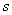in Simple Matching makes no sense because it represents double absence. This may happen when the value of positive and negative do not have equal information (asymmetry). For example, in matching items the customer purchase in a supermarket using Market Basket Analysis , there are more products in the supermarket that the customer does purchase. In this case, the negative value is not important and counting the non-existence in both objects may have no meaningful contribution to the similarity or dissimilarity. Jaccard's coefficient remove thefrom simple matching coefficient to become

Formula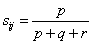Where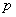= number of variables that positive for both objects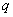= number of variables that positive for the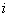th objects and negative for the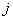th object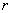= number of variables that negative for theth objects and positive for theth object= number of variables that negative for both objects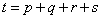= total number of variables

Jaccard's distance can be obtained from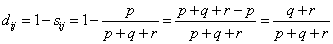Thus,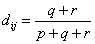To give you more understanding, I provided below an interactive program to compute Jaccard distance and Jaccard Coefficient. Try it yourself your own input values. The examples of computation are given after the program.

Input coordinate values of Object-A and Object-B (the coordinate are binary, number or word), then press "Get Jaccard Cofficient" button to get Jaccard distance and Jaccard Coefficient. The program will directly calculate when you type the input. It will automatically detect whether your inputs are binary or non-binary.

Features Object A Object B

## Example

 Feature of Fruit Sphere shape Sweet Sour Crunchy Object=Apple Yes Yes Yes Yes Object=Banana No Yes No No

The coordinate of Apple is (1,1,1,1) and coordinate of Banana is (0,1,0,0). Because each object is represented by 4 variables, we say that these objects has 4 dimensions.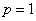,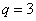and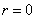,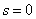.

Jaccard's coefficient between Apple and Banana is 1/4 . Jaccard's distance between Apple and Banana is 3/4.

For non binary data, Jaccard's coefficient can also be computed using set relations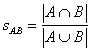Example 2

Suppose we have two sets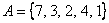and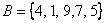.

Then the union is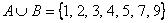and the intersection between two sets is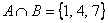. Jaccard's coefficient can be computed based on the number of elements in the intersection set divided by the number of elements in the union set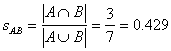Of course, the set formula is also work for binary data, but we need to compute each digit using Boolean algebra. (A and B is True if both true, A or B is false if both False). Intersection set is equivalent to AND, while Union operation is equivalent to OR.

Example 3

Let us use the example above

 A 1 1 1 1 B 0 1 0 0 A and B 0 1 0 0 A or B 1 1 1 1

Sum of all digits can be used to compute Jaccard's coefficient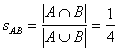the same result as example 1 above.

Note : If your data is binary, you must input as binary in the program above, otherwise it will be detected as non-binary input and you will get incorrect results. For instance, in the Example 1 above, if you input A = (Yes, Yes, Yes, Yes) and B = (No, Yes, No, No), the program will detect as non-binary Jaccard coefficient and produce incorrect Jaccard coefficient of 0.5 (the correct Jaccard coefficient should be 0.25).